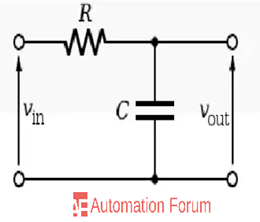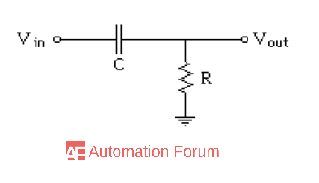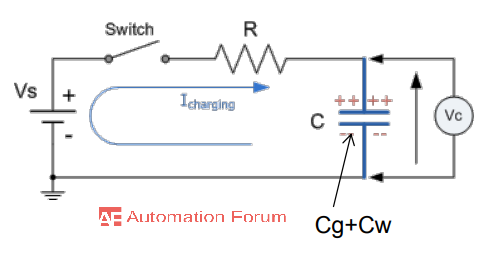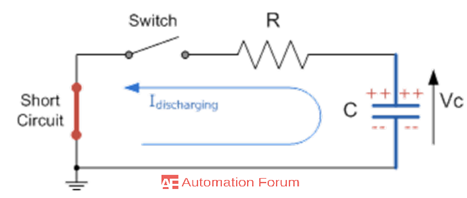# What is RC circuit and its applications

What is RC circuit

An RC circuit can be described as a circuit that has only a single energy storing element and the resistive element is called an RC circuit. RC circuit can be made up of different combinations of capacitors and resistors and this RC circuit has certain frequency response so that it can be used to reduce the amplitude of signals of certain input frequencies while leaving others unaffected. RC circuits can be used to pass low frequencies cutting of high frequencies or they will allow signals to pass which has a certain frequency range.

What is RC lag and lead network

RC lag network can be described as a phase shift circuit in which the output voltage lags the input voltage and the RC lead network is also the phase shift circuit in which the input voltage is led by the output voltage.

What is time constant in an RC circuit

During the charging process the capacitor initial voltage will be zero and the maximum current flow through the circuit is reduced by the resistor and this current is the charging current, and capacitor charge up and the time is taken by the capacitor charge to reach its maximum possible voltage is called as a time constant.

Where are RC- circuit used

RC low pass filter

Low-pass filters can be used to remove the higher frequency signals and then it will allow the low-frequency signals to pass, the signals which have frequencies higher than the cutoff frequency will be removed.An RC low pass filter can be simply made by connecting a single resistor and a capacitor in series. In this type of arrangement the input signal is applied to both the resistor and capacitor together both are in series and the output signal is taken across the capacitor only. This kind of filter is also called the first-order filter because it has only one reactive component in the circuit and it is the capacitor. The reactance of the capacitor varies inversely with the frequency, but the resistor value will be constant as the frequency changes. In case of low frequencies the capacitive reactance will be very high compared to the resistive value and because of that the voltage of the capacitor will be large while compared to the resistor and at high frequencies, this will be different.

RC High pass filterIf the resistor and capacitor are switched in the low pass filter then the voltage across the resistor can be measured. A high pass filter is used to pass the high-frequency signals and removes the low-frequency signals and that’s why it is called a high pass filter. For low-frequency signals the quality is large and the gain is zero while in case of high-frequency signals the quality is small and the gain is close to one, so the signals will be passed.

What is RC charging and discharging circuits

RC charging circuitIn the above figure, the resistor and capacitor are in series forming an RC charging circuit connected across a DC battery supply by through a switch. When the switch is closed current will reach the capacitor by through resistor. The initial voltage through the capacitor will be zero and it will be short-circuited then the maximum current flow through the circuit is restricted by the resistor and this current is called the charging current. After a period of time, the capacitor will be fully charged and the voltage across the capacitor will be equal to the supply voltage and when the capacitor is fully charged no more current will flow into the circuit.

RC discharging circuitIn the above section we discussed the charging of the capacitor through the resistor, now consider the fully charged capacitor is disconnected from the DC battery supply voltage if the battery is removed and replaced by a short circuit and if the switch is closed again then the capacitor will discharge through the resistor. When the charged capacitor is allowed to discharge, the energy that is stored in the capacitor will be transferred to the resistor and the voltage across the capacitor plates will decrease with time.

What are the applications of RC circuits

• They are used in traffic lights
• RC circuits are used in pacemaker
• It can be used in audio equipment
• RC circuits can be used in places were signal filtering is needed
• It can be used as an integrator or differentiator